# Optimization and Machine Learning with Python

This part of the advanced tutorial focuses more on some specific packages. The topics covered in this part are:

• Optimization in Python with OpenOpt.
• Easy and effective Machine Learning in Python with Scikit-Learn.

Prerequisites: Basic and Intermediate tutorials or some experience with Python, Numpy, Scipy, Matplotlib (optional). Basic knowledge in machine learning and optimization.

# 1. Optimization in Python with OpenOpt¶

In this part, we'll learn how to solve optimization problems using the OpenOpt package.

OpenOpt is a free-source package (under the license: BSD) for numerical optimization of a variety of linear, nonlinear problems, least-square problems, network and stochastic problems.

It contains more than 30 different solvers (both free and commercial) including solvers written in C and Fortran. Some solvers are already included in the OpenOpt package (e.g. ralg, gsubg, interalg) and other rely on other packagess (e.g. scipy, IPOPT, ALGENCAN)

In this demonstration we are considering solvers existing in OpenOpt and SciPy. The library workstations have already OpenOpt installed and Scipy is included in the Python distribution. For instructions how to install OpenOpt on other machines go to: http://openopt.org/Install.

Acknowledgements:

## Optimization formulation¶

The general formulation of an optimization problem is to An optimization problem can be expressed in the following way:

• Given: a function $f : A \to R$ from some set $A$ to the set of real numbers
• Want to find a solution $x_0 \in A$ such that $f(x_0)$ is the minimum (or maximum) of $f(x)$ for all $x \in A$.

For example the standard form of a continuous optimization problem is:

$\text{max (or min) } \mathbf{f(x)}$

$\text{subject to } \\$

$\mathbf{lb} \le \mathbf{x} \le \mathbf{ub} \\ \mathbf{A x} \le \mathbf{b} \\ \mathbf{A}_\mathbf{eq} \mathbf{x} = \mathbf{b}_\mathbf{eq} \\ \mathbf{c_i(x) \le 0} \quad \forall i=0,...,I \\ \mathbf{h_j(x) = 0} \quad \forall i=0,...,J \\ \mathbf{x \in R^n}$

where $\mathbf{f, c_i, h_j} :R^n \to R$.

According to the type of the objective function $\mathbf{f(x)}$ and constraints the optimization problem is either linear, quadratic, nonlinear, unconstrained, constrained etc.

### OpenOpt syntax¶

In OpenOpt optimization problems are assigned in the following way:

from openopt import NLP

or other constructor names: LP, MILP, QP, etc according to the problem

Then we use

p = NLP(*args, **kwargs)

to assign the constructor to object p.

Each constructor class has its own arguments

e.g. for NLP it is least f and x0, the objective function and the initial guess.

thus using

p = NLP(objfun, initialguess)

will assign objfun to f and initialguess to x0.

We can use

p.kwargs

to change any kwargs defined in the constructor.

Finally we solve the problem by

r = p.solve(nameOfSolver, otherParameters)

Object r contains all results, i.e. the minimizer, the objective function at solution and output parameters.

### Adding OpenOpt path to PYTHONPATH¶

The best way to see how it works is to go through some examples.

But since OpenOpt is an additional package not included in the Python distribution we use first we need to include its path to the PYTHONPATH.

If you are using a library workstation this can be done in the notebook by running:

In :
import sys
sys.path.append('/Library/Python/2.7/site-packages/openopt-0.5404-py2.7.egg')


On other machines (Linux or Mac) add the following line in the .bashrc (Linux) or .bash_profile (Mac):

export OPENOPT=path-where-the-OOSuite-is

export PYTHONPATH=\$PYTHONPATH:\$OPENOPT

If you don't have such a file then create one.

## Example 1 : Linear problem¶

A linear optimization problem has the following form:

$\text{max (or min) } \mathbf{c}^T\mathbf{x}$

$\text{subject to } \\$

$\mathbf{lb} \le \mathbf{x} \le \mathbf{ub} \\ \mathbf{A x} \le \mathbf{b} \\ \mathbf{A}_\mathbf{eq} \mathbf{x} = \mathbf{b}_\mathbf{eq} \\ \mathbf{x \in R^n}$

Consider the following problem adapted from OpenOpt examples on Linear Problems:

In :
"""
Example:
Let's concider the problem
15x1 + 8x2 + 80x3 -> min        (1)
subjected to
x1 + 2x2 + 3x3 <= 15            (2)
8x1 +  15x2 +  80x3 <= 80       (3)
8x1  + 80x2 + 15x3 <=150        (4)
-100x1 -  10x2 - x3 <= -800     (5)
80x1 + 8x2 + 15x3 = 750         (6)
x1 + 10x2 + 100x3 = 80          (7)
x1 >= 4                         (8)
-8 >= x2 >= -80                 (9)
"""

from numpy import *
from openopt import LP
f = array([15, 8, 80])
A = array([[1, 2, 3], [8, 15, 80], [8, 80, 15], [-100, -10, -1]])
b = array([15, 80, 150, -800])
Aeq = array([[80, 8, 15], [1, 10, 100]])
beq = array([750, 80])

lb = array([4, -80, -inf])
ub = array([inf, -8, inf])
p = LP(f, A=A, Aeq=Aeq, b=b, beq=beq, lb=lb, ub=ub)
#or p = LP(f=f, A=A, Aeq=Aeq, b=b, beq=beq, lb=lb, ub=ub)

#r = p.minimize('glpk') # CVXOPT must be installed
#r = p.minimize('lpSolve') # lpsolve must be installed
r = p.minimize('pclp')
#search for max: r = p.maximize('glpk') # CVXOPT & glpk must be installed

print('objFunValue: %f' % r.ff) # should print 204.48841578
print('x_opt: %s' % r.xf) # should print [ 9.89355041 -8.          1.5010645 ]

------------------------- OpenOpt 0.52 -------------------------
solver: pclp   problem: unnamed    type: LP   goal: minimum
iter   objFunVal   log10(maxResidual)
0  0.000e+00               2.90
1  0.000e+00             -12.94
istop: 1000
Solver:   Time Elapsed = 0.0 	CPU Time Elapsed = 0.01
objFunValue: 204.48842 (feasible, MaxResidual = 1.13687e-13)
objFunValue: 204.488416
x_opt: [ 9.89355041 -8.          1.5010645 ]


We can easily verify that solution satisfies the constraints:

In :
import numpy as np

In :
print np.linalg.norm(np.dot(Aeq,r.xf)- beq)
print np.dot(A,r.xf)- b <= 0

1.21417595911e-13
[ True  True  True  True]


## Example 2: Solving nonlinear equations¶

Solving nonlinear equations can be formulated as minimizing the 2-norm of the vector of equations.

Below is a script solving a system of three equations (from OpenOpt examples on Non-linear least squares).

In :
"""
Let us solve the overdetermined nonlinear equations:
a^2 + b^2 = 15
a^4 + b^4 = 100
a = 3.5

Let us concider the problem as
x**2 + x**2 - 15 = 0
x**4 + x**4 - 100 = 0
x - 3.5 = 0

Now we will solve the one using solver scipy_leastsq
"""
%pylab inline --no-import-all
from openopt import NLLSP
from openopt import NLP

f = lambda x: ((x**2).sum() - 15, (x**4).sum() - 100, x-3.5)
# other possible f assignments:
# f = lambda x: [(x**2).sum() - 15, (x**4).sum() - 100, x-3.5]
#f = [lambda x: (x**2).sum() - 15, lambda x: (x**4).sum() - 100, lambda x: x-3.5]
# f = (lambda x: (x**2).sum() - 15, lambda x: (x**4).sum() - 100, lambda x: x-3.5)
# f = lambda x: asfarray(((x**2).sum() - 15, (x**4).sum() - 100, x-3.5))
def df(x):
r = zeros((3,2))
r[0,0] = 2*x
r[0,1] = 2*x
r[1,0] = 4*x**3
r[1,1] = 4*x**3
r[2,0] = 1
return r

# init esimation of solution - sometimes rather pricise one is very important
x0 = [1.5, 8]

#p = NLLSP(f, x0, diffInt = 1.5e-8, xtol = 1.5e-8, ftol = 1.5e-8)
# or
# p = NLLSP(f, x0)
# or
p = NLLSP(f, x0, xtol = 1.5e-8, ftol = 1.5e-8)

#p.checkdf() # requires DerApproximator to be installed

r = p.solve('scipy_leastsq', plot=1, iprint = 1)

print 'x_opt:', r.xf # 2.74930862,  +/-2.5597651
print 'funcs Values:', p.f(r.xf) # [-0.888904734668, 0.0678251418575, -0.750691380965]
print 'f_opt:', r.ff, '; sum of squares (should be same value):', (p.f(r.xf) ** 2).sum() # 1.35828942657

Populating the interactive namespace from numpy and matplotlib

------------------------- OpenOpt 0.52 -------------------------
solver: scipy_leastsq   problem: unnamed    type: NLLSP
iter   objFunVal
0  1.601e+07
1  1.358e+00
2  1.358e+00
istop: 1000 (Both actual and predicted relative reductions in the sum of squares
are at most 0.000000)
Solver:   Time Elapsed = -0.05 	CPU Time Elapsed = -0.051061
Plotting: Time Elapsed = 0.36 	CPU Time Elapsed = 0.361061
objFunValue: 1.3582894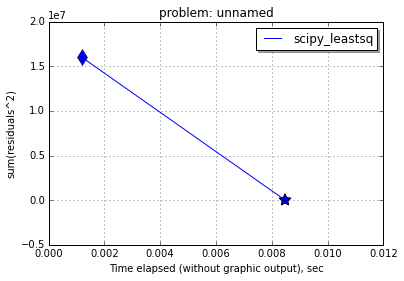x_opt: [ 2.74931062  2.55976255]
funcs Values: [-0.88890684  0.06781965 -0.75068938]
f_opt: 1.3582894266 ; sum of squares (should be same value): 1.3582894266


## Example 3: Least square problem¶

Consider some 2D data. We would like to find a model to the measured data that minimizes the discrepancy between model predictions and the data. This can be formulated by minimizing the sum of the squares of the difference between values of model and measured data.

First create some data and plot them:

In :
import matplotlib.pyplot as plt

In :
n = 200
X = np.linspace(0,2,n)
m = lambda X : 0.8*np.exp(-1.5*X)+1.2*np.exp(-0.8*X)
perturb = 0.2*np.random.uniform(0,1,n)
Y = m(X)*(1.0+perturb)

In :
plt.figure(figsize=(15,10), dpi=100) # changing figure's shape
plt.plot(X,Y,'.')
plt.xlabel('$X$',fontsize=16) # horizontal axis name
plt.ylabel('Y',fontsize=16) # vertical axis name
plt.title('Sample data',fontsize=18) # title
plt.grid(True) # enabling grid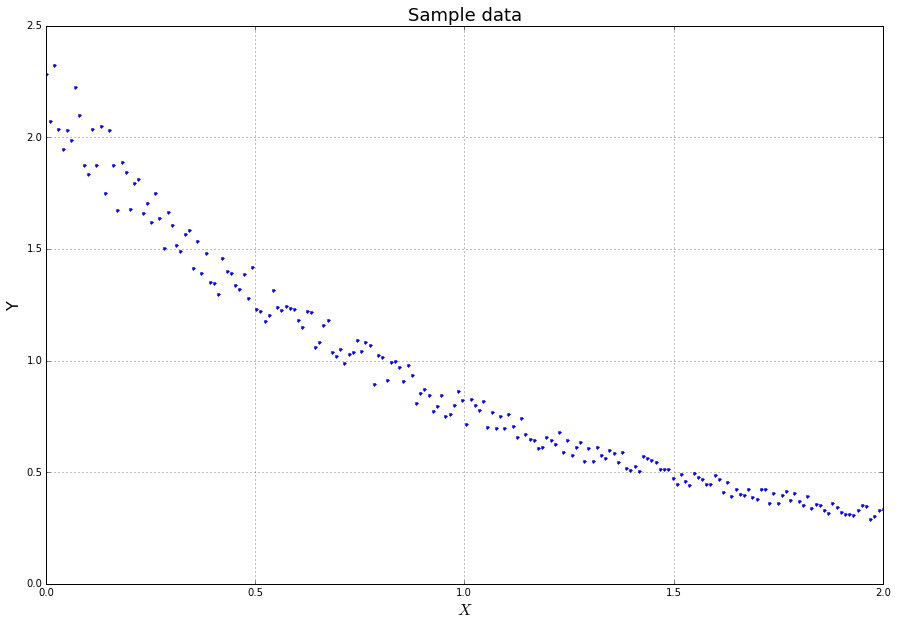Now let's solve the least square problem:

In :
"""
In the DFP example we will search for z=(a, b, c, d)
that minimizes Sum_i || F(z, X_i) - Y_i ||^2
for the function
F(x) = a*exp(-b*x) + c*exp(-d*x)
"""
from openopt import DFP

f = lambda z, X: z*exp(-z*X) + z*exp(-z*X)
initEstimation = [0.2, 0.9, 1.9, 0.9]
lb = [-np.inf, -np.inf, -np.inf, -np.inf]
ub = [np.inf, np.inf, np.inf, np.inf]
p = DFP(f, initEstimation, X, Y, lb=lb, ub=ub)

p.df = lambda z, X: [exp(-z*X), -z*z*exp(-z*X), exp(-z*X), -z*z*exp(-z*X)]

r = p.solve('nlp:ralg', plot=1, iprint = 10)
f = lambda z, X: z*exp(-z*X) + z*exp(-z*X)
print('solution: '+str(r.xf)+'\n||residuals||^2 = '+str(r.ff))

------------------------- OpenOpt 0.52 -------------------------
solver: ralg   problem: unnamed    type: DFP
iter   objFunVal
0  1.147e+00
10  1.460e+00
20  6.557e-01
30  6.381e-01
40  6.384e-01
50  6.386e-01
60  6.387e-01
70  6.384e-01
80  6.379e-01
90  6.370e-01
100  6.380e-01
109  6.367e-01
istop: 4 (|| F[k] - F[k-1] || < ftol)
Solver:   Time Elapsed = 2.59 	CPU Time Elapsed = 2.608965
Plotting: Time Elapsed = 2.36 	CPU Time Elapsed = 2.341035
objFunValue: 0.63670925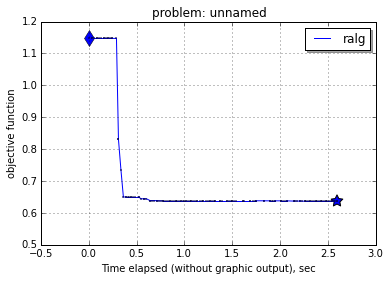solution: [ 0.33837813  2.26118336  1.86071125  0.90294692]
||residuals||^2 = 0.636709253815

In :
fun = lambda z, X: z*exp(-z*X) + z*exp(-z*X)

plt.figure(figsize=(15,10), dpi=100) # changing figure's shape
plt.plot(X,Y,'.')
plt.plot(X,fun(r.xf,X),'r-',linewidth=1.5)
plt.xlabel('$X$',fontsize=16) # horizontal axis name
plt.ylabel('Y',fontsize=16) # vertical axis name
plt.title('Sample data',fontsize=18) # title
plt.grid(True) # enabling grid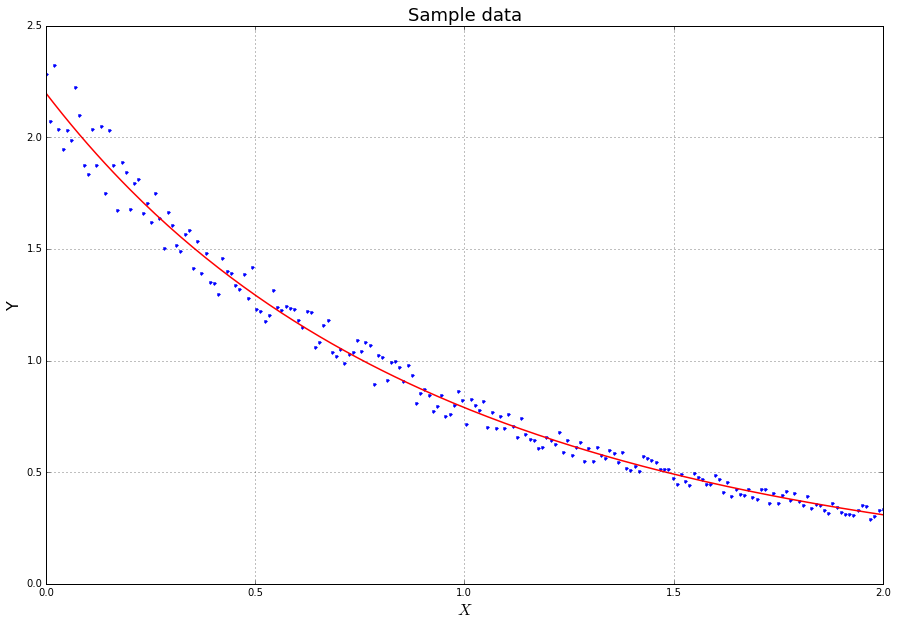## Example 4: Nonlinear problem¶

Finally, the following is an example of a non-linear problem (from OpenOpt non-linear examples) as described at the beginning of this part.

In :
"""
Example:
(x0-5)^2 + (x2-5)^2 + ... +(x149-5)^2 -> min

subjected to

# lb<= x <= ub:
x4 <= 4
8 <= x5 <= 15

# Ax <= b
x0+...+x149 >= 825
x9 + x19 <= 3
x10+x11 <= 9

# Aeq x = beq
x100+x101 = 11

# c(x) <= 0
2*x0^4-32 <= 0
x1^2+x2^2-8 <= 0

# h(x) = 0
(x-1)**6 = 0
(x-1.5)**6 = 0
"""

from openopt import NLP
from numpy import cos, arange, ones, asarray, zeros, mat, array
N = 150

# objective function:
f = lambda x: ((x-5)**2).sum()

df = lambda x: 2*(x-5)

# start point (initial estimation)
x0 = 8*cos(arange(N))

# c(x) <= 0 constraints
c = [lambda x: 2* x **4-32, lambda x: x**2+x**2 - 8]

# dc(x)/dx: non-lin ineq constraints gradients (optional):
dc0 = lambda x: [8 * x**3] + *(N-1)
dc1 = lambda x: [0, 2 * x,  2 * x] + *(N-3)
dc = [dc0, dc1]

# h(x) = 0 constraints
def h(x):
return (x[N-1]-1)**6, (x[N-2]-1.5)**6
# other possible return types: numpy array, matrix, Python list, tuple
# or just h = lambda x: [(x-1)**6, (x-1.5)**6]

# dh(x)/dx: non-lin eq constraints gradients (optional):
def dh(x):
r = zeros((2, N))
r[0, -1] = 6*(x[N-1]-1)**5
r[1, -2] = 6*(x[N-2]-1.5)**5
return r

# lower and upper bounds on variables
lb = -6*ones(N)
ub = 6*ones(N)
ub = 4
lb, ub = 8, 15

# general linear inequality constraints
A = zeros((3, N))
A[0, 9] = 1
A[0, 19] = 1
A[1, 10:12] = 1
A = -ones(N)
b = [7, 9, -825]

# general linear equality constraints
Aeq = zeros(N)
Aeq[100:102] = 1
beq = 11

# required constraints tolerance, default for NLP is 1e-6
contol = 1e-7

# If you use solver algencan, NB! - it ignores xtol and ftol; using maxTime, maxCPUTime, maxIter, maxFunEvals, fEnough is recommended.
# Note that in algencan gtol means norm of projected gradient of  the Augmented Lagrangian
# so it should be something like 1e-3...1e-5
gtol = 1e-7 # (default gtol = 1e-6)

# Assign problem:
# 1st arg - objective function
# 2nd arg - start point
p = NLP(f, x0, df=df,  c=c,  dc=dc, h=h,  dh=dh,  A=A,  b=b,  Aeq=Aeq,  beq=beq,
lb=lb, ub=ub, gtol=gtol, contol=contol, iprint = 50, maxIter = 10000, maxFunEvals = 1e7, name = 'NLP_1')

#optional: graphic output, requires pylab (matplotlib)
p.plot = True

solver = 'ralg'
#solver = 'scipy_slsqp'

# solve the problem
r = p.solve(solver, plot=1) # string argument is solver name

# r.xf and r.ff are optim point and optim objFun value
# r.ff should be something like 132.05
print r.ff

------------------------- OpenOpt 0.52 -------------------------
solver: ralg   problem: NLP_1    type: NLP   goal: minimum
iter   objFunVal   log10(maxResidual)
0  8.596e+03               5.73
50  3.033e+02               3.79
100  2.047e+02               4.15
150  1.863e+02               3.89
200  1.685e+02               4.06
250  1.798e+02               4.21
300  1.670e+02               3.90
350  1.652e+02               4.18
400  1.524e+02               3.73
450  1.713e+02               3.90
500  1.518e+02               4.11
550  1.633e+02               3.87
600  1.603e+02               3.76
650  1.553e+02               3.19
700  1.632e+02               2.63
750  2.084e+02               0.98
800  2.076e+02              -1.99
850  1.479e+02              -3.01
900  1.363e+02              -3.56
950  1.321e+02              -7.10
1000  1.372e+02              -7.09
1050  1.372e+02              -7.08
1100  1.372e+02              -7.08
1144  1.321e+02              -7.10
istop: 3 (|| X[k] - X[k-1] || < xtol)
Solver:   Time Elapsed = 4.27 	CPU Time Elapsed = 4.288232
Plotting: Time Elapsed = 5.99 	CPU Time Elapsed = 5.971768
objFunValue: 132.06825 (feasible, MaxResidual = 7.98482e-08)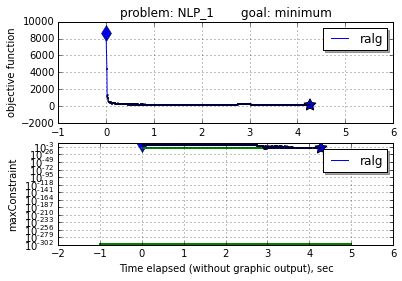132.068251573


# 1. Machine Learning in Python¶

Machine learning is a hot and popular topic nowadays. It is about building programs with tunable parameters that are adjusted automatically by adapting to previously seen data. It intervenes literally every sphere of our life ranging from science, engineering, and industry to economics, sociology, and even personal relationships. So if it is applicable everywhere, we need to have handy tools for doing machine learning.

In :
# Start pylab inline mode, so figures will appear in the notebook
%pylab inline

Populating the interactive namespace from numpy and matplotlib


### 1.1. Quick Overview of Scikit-Learn¶

In :
# Load a dataset already available in scikit-learn
from sklearn import datasets
type(digits)

Out:
sklearn.datasets.base.Bunch
In :
digits.data

Out:
array([[  0.,   0.,   5., ...,   0.,   0.,   0.],
[  0.,   0.,   0., ...,  10.,   0.,   0.],
[  0.,   0.,   0., ...,  16.,   9.,   0.],
...,
[  0.,   0.,   1., ...,   6.,   0.,   0.],
[  0.,   0.,   2., ...,  12.,   0.,   0.],
[  0.,   0.,  10., ...,  12.,   1.,   0.]])
In :
digits.target

Out:
array([0, 1, 2, ..., 8, 9, 8])
In :
from sklearn import svm
clf = svm.SVC(gamma=0.001, C=100.)
clf.fit(digits.data[:-1], digits.target[:-1])

print "Original label:", digits.target
print "Predicted label:", clf.predict(digits.data)

plt.figure(figsize=(3, 3))
plt.imshow(digits.images, interpolation='nearest', cmap=plt.cm.binary)

Original label: 5
Predicted label: 

Out:
<matplotlib.image.AxesImage at 0x1110cc7d0>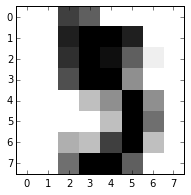### 1.2. Data representation in Scikit-learn¶

Data in scikit-learn, with very few exceptions, is assumed to be stored as a two-dimensional array, of size [n_samples, n_features], and most of machine learning algorithms implemented in scikit-learn expect data to be stored this way.

• n_samples: The number of samples: each sample is an item to process (e.g. classify). A sample can be a document, a picture, a sound, a video, an astronomical object, a row in database or CSV file, or whatever you can describe with a fixed set of quantitative traits.
• n_features: The number of features or distinct traits that can be used to describe each item in a quantitative manner. Features are generally real-valued, but may be boolean or discrete-valued in some cases.

The number of features must be fixed in advance. However it can be very high dimensional (e.g. millions of features) with most of them being zeros for a given sample. This is a case where scipy.sparse matrices can be useful, in that they are much more memory-efficient than numpy arrays.

#### 1.2.1. Example: iris dataset¶

To understand these simple concepts better, let's work with an example: iris-dataset.

In :
# Don't know what iris is? Run this code to generate some pictures.
from IPython.core.display import Image, display
display(Image(filename='figures/iris_setosa.jpg'))
print "Iris Setosa\n"

display(Image(filename='figures/iris_versicolor.jpg'))
print "Iris Versicolor\n"

display(Image(filename='figures/iris_virginica.jpg'))
print "Iris Virginica"Iris SetosaIris VersicolorIris Virginica

In :
# Load the dataset from scikit-learn
type(iris) # The resulting dataset is a Bunch object

Out:
sklearn.datasets.base.Bunch
In :
iris.keys()

Out:
['target_names', 'data', 'target', 'DESCR', 'feature_names']
In :
print iris.DESCR

Iris Plants Database

Notes
-----
Data Set Characteristics:
:Number of Instances: 150 (50 in each of three classes)
:Number of Attributes: 4 numeric, predictive attributes and the class
:Attribute Information:
- sepal length in cm
- sepal width in cm
- petal length in cm
- petal width in cm
- class:
- Iris-Setosa
- Iris-Versicolour
- Iris-Virginica
:Summary Statistics:
============== ==== ==== ======= ===== ====================
Min  Max   Mean    SD   Class Correlation
============== ==== ==== ======= ===== ====================
sepal length:   4.3  7.9   5.84   0.83    0.7826
sepal width:    2.0  4.4   3.05   0.43   -0.4194
petal length:   1.0  6.9   3.76   1.76    0.9490  (high!)
petal width:    0.1  2.5   1.20  0.76     0.9565  (high!)
============== ==== ==== ======= ===== ====================
:Missing Attribute Values: None
:Class Distribution: 33.3% for each of 3 classes.
:Creator: R.A. Fisher
:Donor: Michael Marshall (MARSHALL%[email protected])
:Date: July, 1988

This is a copy of UCI ML iris datasets.
http://archive.ics.uci.edu/ml/datasets/Iris

The famous Iris database, first used by Sir R.A Fisher

This is perhaps the best known database to be found in the
pattern recognition literature.  Fisher's paper is a classic in the field and
is referenced frequently to this day.  (See Duda & Hart, for example.)  The
data set contains 3 classes of 50 instances each, where each class refers to a
type of iris plant.  One class is linearly separable from the other 2; the
latter are NOT linearly separable from each other.

References
----------
- Fisher,R.A. "The use of multiple measurements in taxonomic problems"
Annual Eugenics, 7, Part II, 179-188 (1936); also in "Contributions to
Mathematical Statistics" (John Wiley, NY, 1950).
- Duda,R.O., & Hart,P.E. (1973) Pattern Classification and Scene Analysis.
(Q327.D83) John Wiley & Sons.  ISBN 0-471-22361-1.  See page 218.
- Dasarathy, B.V. (1980) "Nosing Around the Neighborhood: A New System
Structure and Classification Rule for Recognition in Partially Exposed
Environments".  IEEE Transactions on Pattern Analysis and Machine
Intelligence, Vol. PAMI-2, No. 1, 67-71.
- Gates, G.W. (1972) "The Reduced Nearest Neighbor Rule".  IEEE Transactions
on Information Theory, May 1972, 431-433.
- See also: 1988 MLC Proceedings, 54-64.  Cheeseman et al"s AUTOCLASS II
conceptual clustering system finds 3 classes in the data.
- Many, many more ...


In :
# Number of samples and features
iris.data.shape
print "Number of samples:", iris.data.shape
print "Number of features:", iris.data.shape
print iris.data

Number of samples: 150
Number of features: 4
[ 5.1  3.5  1.4  0.2]

In :
# Target (label) names
print iris.target_names

['setosa' 'versicolor' 'virginica']

In :
# Visualize the data
x_index = 0
y_index = 1

# this formatter will label the colorbar with the correct target names
formatter = plt.FuncFormatter(lambda i, *args: iris.target_names[int(i)])

plt.figure(figsize=(7, 5))
plt.scatter(iris.data[:, x_index], iris.data[:, y_index], c=iris.target)
plt.colorbar(ticks=[0, 1, 2], format=formatter)
plt.xlabel(iris.feature_names[x_index])
plt.ylabel(iris.feature_names[y_index])

Out:
<matplotlib.text.Text at 0x110c5a890>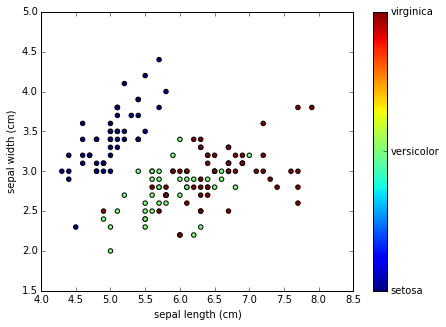Quick Exercise: Change x_index and y_index and find the 2D projection of the data which maximally separate all three classes.

In :
# your code goes here


#### 1.2.2. Other available datasets¶

Scikit-learn makes available a host of datasets for testing learning algorithms. They come in three flavors:

• Packaged Data: these small datasets are packaged with the scikit-learn installation, and can be downloaded using the tools in sklearn.datasets.load_*
• Downloadable Data: these larger datasets are available for download, and scikit-learn includes tools which streamline this process. These tools can be found in sklearn.datasets.fetch_*
• Generated Data: there are several datasets which are generated from models based on a random seed. These are available in the sklearn.datasets.make_*
In :
# Where the downloaded datasets via fetch_scripts are stored
from sklearn.datasets import get_data_home
get_data_home()

Out:
'/Users/hadjimy/scikit_learn_data'
In :
# How to fetch an external dataset
from sklearn import datasets


Now you can type datasets.fetch_<TAB> and a list of available datasets will pop up.

#### Exercise:¶

Fetch the dataset olivetti_faces. Visualize various 2D projections for the data. Find the best in terms of separability.

In :
from sklearn.datasets import fetch_olivetti_faces

In :
# your code goes here
# fetch the faces data
# Use a script like above to plot the faces image data.
# Hint: plt.cm.bone is a good colormap for this data.

In :
#%load solutions/sklearn_vis_data.py


### 1.3. Linear regression and classification¶

In this section, we explain how to use scikit-learn library for regression and classification. We discuss Estimator class - the base class for many of the scikit-learn models - and work through some examples.

In :
from sklearn.datasets import load_boston
print data.DESCR

Boston House Prices dataset

Notes
------
Data Set Characteristics:

:Number of Instances: 506

:Number of Attributes: 13 numeric/categorical predictive

:Median Value (attribute 14) is usually the target

:Attribute Information (in order):
- CRIM     per capita crime rate by town
- ZN       proportion of residential land zoned for lots over 25,000 sq.ft.
- INDUS    proportion of non-retail business acres per town
- CHAS     Charles River dummy variable (= 1 if tract bounds river; 0 otherwise)
- NOX      nitric oxides concentration (parts per 10 million)
- RM       average number of rooms per dwelling
- AGE      proportion of owner-occupied units built prior to 1940
- DIS      weighted distances to five Boston employment centres
- TAX      full-value property-tax rate per $10,000 - PTRATIO pupil-teacher ratio by town - B 1000(Bk - 0.63)^2 where Bk is the proportion of blacks by town - LSTAT % lower status of the population - MEDV Median value of owner-occupied homes in$1000's

:Missing Attribute Values: None

:Creator: Harrison, D. and Rubinfeld, D.L.

This is a copy of UCI ML housing dataset.
http://archive.ics.uci.edu/ml/datasets/Housing

This dataset was taken from the StatLib library which is maintained at Carnegie Mellon University.

The Boston house-price data of Harrison, D. and Rubinfeld, D.L. 'Hedonic
prices and the demand for clean air', J. Environ. Economics & Management,
vol.5, 81-102, 1978.   Used in Belsley, Kuh & Welsch, 'Regression diagnostics
...', Wiley, 1980.   N.B. Various transformations are used in the table on
pages 244-261 of the latter.

The Boston house-price data has been used in many machine learning papers that address regression
problems.

**References**

- Belsley, Kuh & Welsch, 'Regression diagnostics: Identifying Influential Data and Sources of Collinearity', Wiley, 1980. 244-261.
- Quinlan,R. (1993). Combining Instance-Based and Model-Based Learning. In Proceedings on the Tenth International Conference of Machine Learning, 236-243, University of Massachusetts, Amherst. Morgan Kaufmann.
- many more! (see http://archive.ics.uci.edu/ml/datasets/Housing)


In :
# Plot histogram for real estate prices
plt.hist(data.target)
plt.xlabel('price ($1000s)') plt.ylabel('count')  Out: <matplotlib.text.Text at 0x11173cf90>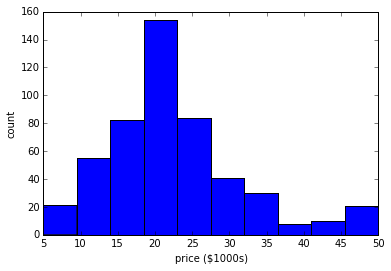Quick Exercise: Try sctter-plot several 2D projections of the data. What you can infer from the plots? In : # your code goes here  Every algorithm is exposed in scikit-learn via an Estimator object. For instance a linear regression is: In : from sklearn.linear_model import LinearRegression clf = LinearRegression() clf.fit(data.data, data.target)  Out: LinearRegression(copy_X=True, fit_intercept=True, normalize=False) In : predicted = clf.predict(data.data)  In : # Plot the predicted price vs. true price plt.scatter(data.target, predicted) plt.plot([0, 50], [0, 50], '--k') plt.axis('tight') plt.xlabel('True price ($1000s)')
plt.ylabel('Predicted price (\$1000s)')

Out:
<matplotlib.text.Text at 0x11177ec50>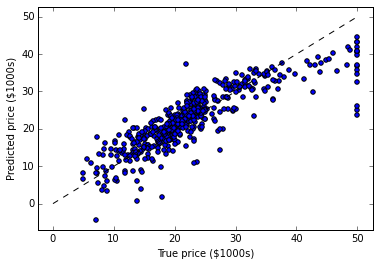Examples of regression type problems in machine learning:

• Sales: given consumer data, predict how much they will spend
• Collaborative Filtering: given a collection of user-ratings for movies, predict preferences for other movies & users.
• Astronomy: given observations of galaxies, predict their mass or redshift

Another example of regularized linear regression on a synthetic data set.

In :
import numpy as np
from sklearn.linear_model import LinearRegression

rng = np.random.RandomState(0)
x = 2 * rng.rand(100) - 1

f = lambda t: 1.2 * t ** 2 + .1 * t ** 3 - .4 * t ** 5 - .5 * t ** 9
y = f(x) + .4 * rng.normal(size=100)

plt.figure(figsize=(7, 5))
plt.scatter(x, y, s=4)

Out:
<matplotlib.collections.PathCollection at 0x1118510d0>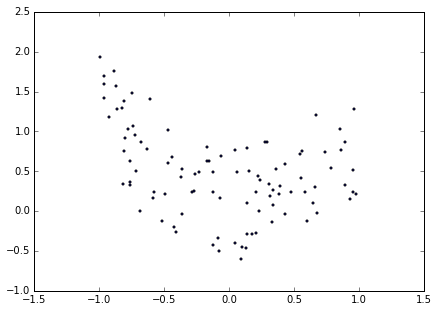In :
x_test = np.linspace(-1, 1, 100)

for k in [4, 9]:
X = np.array([x**i for i in range(k)]).T
X_test = np.array([x_test**i for i in range(k)]).T
order4 = LinearRegression()
order4.fit(X, y)
plt.plot(x_test, order4.predict(X_test), label='%i-th order'%(k))

plt.legend(loc='best')
plt.axis('tight')
plt.title('Fitting a 4th and a 9th order polynomial')

Out:
<matplotlib.text.Text at 0x111871050>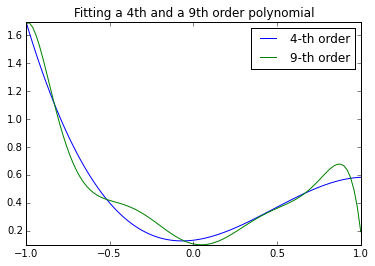In :
# Let's look at the ground truth
plt.figure()
plt.scatter(x, y, s=4)
plt.plot(x_test, f(x_test), label="truth")
plt.axis('tight')
plt.title('Ground truth (9th order polynomial)')

Out:
<matplotlib.text.Text at 0x111ae7dd0>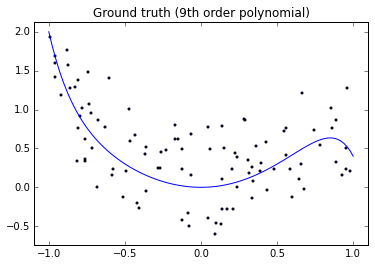Let's use Ridge regression (with l1-norm regularization)

In :
from sklearn.linear_model import Ridge

for k in :
X = np.array([x**i for i in range(k)]).T
X_test = np.array([x_test**i for i in range(k)]).T
order4 = Ridge(alpha=0.3)
order4.fit(X, y)
plt.plot(x_test, order4.predict(X_test), label='%i-th order'%(k))
plt.plot(x_test, f(x_test), label="truth")
plt.scatter(x, y, s=4)
plt.legend(loc='best')
plt.axis('tight')
plt.title('Fitting a 4th and a 9th order polynomial')

Out:
<matplotlib.text.Text at 0x111c2dad0>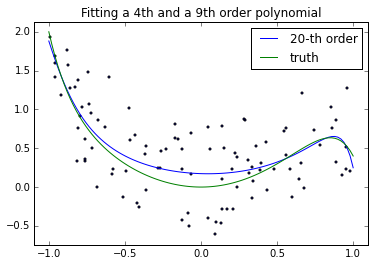In :
plt.ylim?


#### Exercise:¶

For this exercise, you need to classify iris data with nearest neighbors classifier and with linear support vector classifier (LinearSVC). In order to measure the performance, calculate the accuracy or just use clf.score function built-in into the Estimator object.

In [ ]:
from __future__ import division  # turn off division truncation -- division will be floating point by default
from sklearn.neighbors import KNeighborsClassifier
from sklearn.svm import LinearSVC

# Splitting the data into train and test sets
indices = np.random.permutation(range(iris.data.shape))
train_sz = floor(0.8*iris.data.shape)
X, y = iris.data[indices[:train_sz],:], iris.target[indices[:train_sz]]
Xt, yt = iris.data[indices[train_sz:],:], iris.target[indices[train_sz:]]


In :
#%load solutions/iris_classification.py


### 1.4. Approaches to validation and testing¶

It is usually an arduous and time consuming task to write cross-validation or other testing wrappers for your models. Scikit-learn allows you validate your models out-of-the-box. Let's have a look at how to do this.

In :
from sklearn import cross_validation
X, Xt, y, yt = cross_validation.train_test_split(iris.data, iris.target, test_size=0.4, random_state=0)
print X.shape, y.shape
print Xt.shape, yt.shape

(90, 4) (90,)
(60, 4) (60,)

In [ ]:
# Cross-validating your models in one line
scores = cross_validation.cross_val_score(svc, iris.data, iris.target, cv=5,)
print scores
print scores.mean()

In [ ]:
cross_validation.cross_val_score?

In [ ]:
# Calculate F1-measure (or any other score)
from sklearn import metrics
f1_scores = cross_validation.cross_val_score(svc, iris.data, iris.target, cv=5, scoring='f1')
print f1_scores
print f1_scores.mean()


Quick Exercise: Do the same CV-evaluation of the KNN classifier. Find the best number of nearest neighbors.

In [ ]:



### 1.5. What to learn next?¶

If you would like to start using Scikit-learn for your machine learning tasks, the most right way is to jump into it and refer to the documentation from time to time. When you acquire enough skills in it, you can go and check out the following resources: